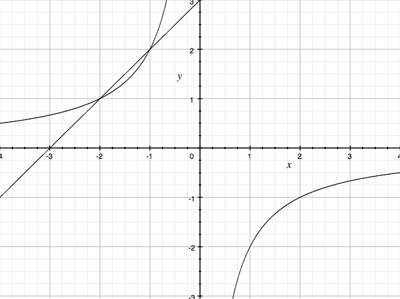SEARCH HOMEMath Central Quandaries & QueriesQuestion from Megan: How do i find the interception points of xy=-2 and y=x+3? Many thanks!Hi Megan,

Since $y = x + 3$ you can substitute this into the first equation to get

$xy = x(x+3) = -2.$

Simplify and solve the resulting quadratic equation for $x.$ Find the $y$ value for each of the resulting $x$ values using $y = x + 3.$

I am a strong believer in using a graphic approach to see if my answer makes sense In this case I gotPenny* Registered trade mark of Imperial Oil Limited. Used under license.Math Central is supported by the University of Regina and the Imperial Oil Foundation.